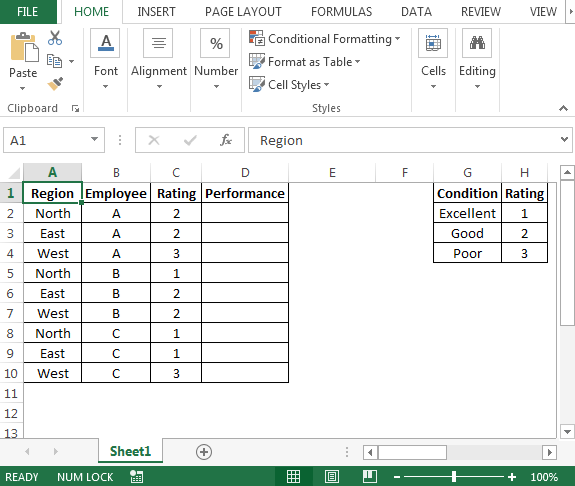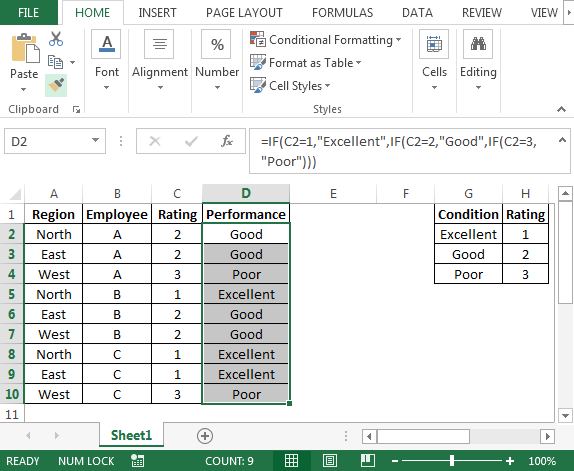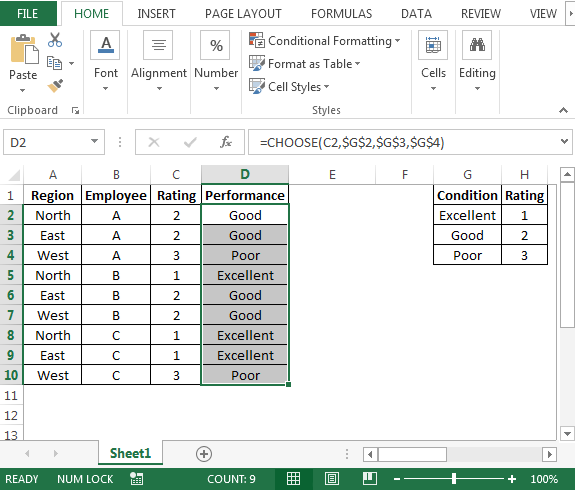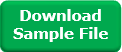Nested IF statement in Microsoft Excel

In case you want to learn Excel IF function then this article is for you. In this article, we will focus on describing the use of IF function in multiple conditions.

IF function use to check the specified condition is True or False. If the condition is true then we get the preset output, and if the condition returns FALSE then it returns specified preset value.

Syntax = IF(logical_test,value_if_true,value_if_false)

The alternate function of IF function is Choose. Choose will take less time than in comparison of IF function to get result.

Choose function used to returns the character specified by the code number from the character set for your computer. Also it  will return a value from a list of values based on a given index number. Choose function uses index_num to return a value from a list.

Syntax = CHOOSE(index_num,value1,value2,…)

Following is the snapshot of data we have:• Employee data is placed in Column A, B & C.
• We need a formula to check the value in column C to meet the following conditions in column G & H, respectively.
• In cell D2, the  formula is
• =IF(C2=1,”Excellent”,IF(C2=2,”Good”,IF(C2=3,”Poor”)))• In Nested IF function, the thumb rule is to write condition from high to low or low to high.
• In this way, using Nested IF function, we can calculate the result, meeting multiple criteria at one time.

Now we will learn how to use CHOOSE function to replace IF function.

• In cell D2, the formula is
• =CHOOSE(C2,\$G\$2,\$G\$3,\$G\$4)In this way, we have learnt both IF & CHOOSE functions.Terms and Conditions of use

The applications/code on this site are distributed as is and without warranties or liability. In no event shall the owner of the copyrights, or the authors of the applications/code be liable for any loss of profit, any problems or any damage resulting from the use or evaluation of the applications/code.